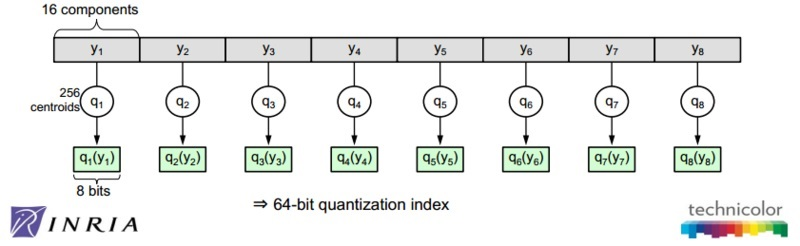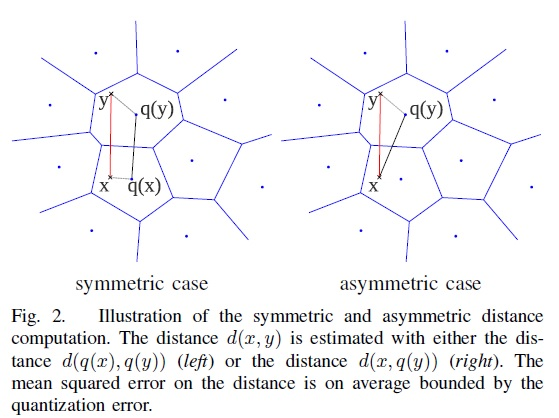05 August 2017

## 1. 引言

Product quantization，国内有人直译为乘积量化，这里的乘积是指笛卡尔积（Cartesian product），意思是指把原来的向量空间分解为若干个低维向量空间的笛卡尔积，并对分解得到的低维向量空间分别做量化（quantization）。这样每个向量就能由多个低维空间的量化code组合表示。为简洁描述起见，下文用PQ作为product quantization的简称。

The idea is to decomposes the space into a Cartesian product of low dimensional subspaces and to quantize each subspace separately. A vector is represented by a short code composed of its subspace quantization indices.

2011年，Herve Jegou等学者在PAMI上发表了PQ方法的第一篇正式paper，用于解决相似搜索问题（similarity search）或者也可以说是近邻搜索（nearest neighbor search）问题。其实这几位作者在2009年的INRIA（即法国国家信息与自动化研究所）的技术报告上已经发表PQ方法。这里插一段题外话，的一作Herve Jegou和二作Matthijs Douze均在2015年跳槽去了Facebook AI research，并在今年3月份合作开源了Faiss相似搜索工具。

PQ是一种量化（quantization）方法，本质上是数据的一种压缩表达方法（其实通信学科的一个主要研究工作就是研究信号的压缩表达），所以该方法除了可以用在相似搜索外，还可以用于模型压缩，特别是深度神经网络的模型压缩上。由于相似搜索不仅要考虑如何量化的问题，还要考虑如何检索（search）的问题，而模型压缩可能更主要的是考虑如何量化的问题，不用太关注如何检索这个问题，所以这篇文章会主要站在相似搜索上的应用来介绍PQ方法。至于模型压缩，可以找找近几年研究神经网络模型压缩的paper或者一些互联网公司（比如百度, Snap等）发出的一些资料。

## 2. 相似搜索的若干种方法

• 基于树的方法
• KD树是其下的经典算法。一般而言，在空间维度比较低时，KD树的查找性能还是比较高效的；但当空间维度较高时，该方法会退化为暴力枚举，性能较差，这时一般会采用下面的哈希方法或者矢量量化方法。
• 哈希方法
• LSH(Locality-Sensitive Hashing)是其下的代表算法。文献是一篇非常好的LSH入门资料。
• 对于小数据集和中规模的数据集(几个million-几十个million)，基于LSH的方法的效果和性能都还不错。这方面有2个开源工具FALCONN和NMSLIB。
• 矢量量化方法
• 矢量量化方法，即vector quantization。在矢量量化编码中，关键是码本的建立和码字搜索算法。比如常见的聚类算法，就是一种矢量量化方法。而在相似搜索中，向量量化方法又以PQ方法最为典型。
• 对于大规模数据集(几百个million以上)，基于矢量量化的方法是一个明智的选择，可以用用Faiss开源工具。

## 3. Product Quantization算法的核心

Quantization is a destructive process which has been extensively studied in information theory. Its purpose is to reduce the cardinality of the representation space, in particular when the input data is real-valued. Formally, a quantizer is a function $$q$$ mapping a $$D$$-dimensional vector $$x\in{R^D}$$ to a vector $$q(x)\in{C}={c_{i}; i\in{I}}$$, where the index set $$I$$ is from now on assumed to be finite: $$I = 0, \cdots , k-1$$. The reproduction values $$c_i$$ are called $$\color{red}{centroids}$$. The set of reproduction values $$C$$ is the $$\color{red}{codebook}$$ of size $$k$$.

The strength of a product quantizer is to produce a large set of centroids from several small sets of centroids: those associated with the subquantizers. When learning the subquantizers using Lloyd’s algorithm, a limited number of vectors is used, but the codebook is, to some extent, still adapted to the data distribution to represent. The complexity of learning the quantizer is m times the complexity of performing k-means clustering with $$k^*$$ centroids of dimension $$D^*$$.SDC算法：先用PQ量化器对$$x$$和$$y$$表示为对应的中心点$$q(x)$$和$$q(y)$$，然后用公式1来近似$$d(x,y)$$。

\begin{equation}\hat{d}(x,y)=d(q(x),q(y))=\sqrt{\sum_{j} d(q_j(x), q_j(y))^2}\end{equation}

1. 为提高计算速度，一般会提前算好$$d(c_{j,i}, c_{j,i’})^2$$，然后在检索时就是查表，以O(1)的复杂度查出结果。
2. $$\hat{d}(x,y)$$是$$d(x,y)$$的近似计算，一般会先用相似计算方法选出top N近邻，然后再做rerank以拿到最终的近邻排序结果。

ADC算法：只对$$y$$表示为对应的中心点$$q(y)$$，然后用公式2来近似$$d(x,y)$$。

\begin{equation}\widetilde{d}(x,y)=d(x,q(y))=\sqrt{\sum_{j} d(u_j(x), q_j(u_j(y)))^2}\end{equation}

1. 为提高计算速度，一般会在检索前提前算好$$d(u_j(x), c_{j,i})^2 : j=1,\cdots, m, i=1,\cdots, k^*$$，然后在检索时就是查表，以O(1)的复杂度查出结果。
2. $$\widetilde{d}(x,y)$$也是$$d(x,y)$$的近似计算，与SDC类似，一般会先用相似计算方法选出top N近邻，然后再做rerank以拿到最终的近邻排序结果。## 4. Product Quantization算法的改进

The energy of the residual vector is small compared to that of the vector itself.1. 考虑到在coarse quantization中，$$x$$和它的近邻不一定落在同一个簇中，所以在查询coarse quantization时，会同时取出$$w$$个倒排链。
2. 对取出的每个倒排链，还是用第3节介绍的PQ算法把近邻给找出。
3. 考虑当$$n>k^*D^*$$时，朴素的ADC算法的复杂度是$$O(n \times m)$$，而IVFADC算法的复杂度会降低为$$O((n \times w / k’) \times m)$$。

 Faiss